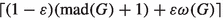# On the Density of Critical Graphs with No Large Cliques

## Abstract

A graph G is k-critical if $$\chi (G) = k$$ and every proper subgraph of G is $$(k - 1)$$-colorable, and if L is a list assignment for G, then G is L-critical if G is not L-colorable but every proper subgraph of G is. In 2014, Kostochka and Yancey proved a lower bound on the average degree of an n-vertex k-critical graph tending to $$k - \frac{2}{k - 1}$$ for large n that is tight for infinitely many values of n, and they asked how their bound may be improved for graphs not containing a large clique. Answering this question, we prove that there exists some $$\varepsilon > 0$$ for which the following holds. If k is sufficiently large and G is a $$K_{\omega + 1}$$-free L-critical graph where $$\omega \le k - \log ^{10}k$$ and L is a list assignment for G such that $$|L(v)| = k - 1$$ for all $$v\in V(G)$$, then the average degree of G is at least $$(1 + \varepsilon )(k - 1) - \varepsilon \omega - 1$$. This result implies that for some $$\varepsilon > 0$$, for every graph G satisfying $$\omega (G) \le \textrm{mad}(G) - \log ^{10}\textrm{mad}(G)$$ where $$\omega (G)$$ is the size of the largest clique in G and $$\textrm{mad}(G)$$ is the maximum average degree of G, the list-chromatic number of G is at most.

This is a preview of subscription content, log in via an institution to check access.

Price excludes VAT (USA)
Tax calculation will be finalised during checkout.

## References

1. Appel, K., Haken, W.: Every planar map is four colorable. Bull. Am. Math. Soc. 82(5), 711–712 (1976)

2. Bonamy, M., Perrett, T., Postle, L.: Colouring graphs with sparse neighbourhoods: bounds and applications. J. Comb. Theory Ser. B 155, 278–317 (2022)

3. Brooks, R.L.: On colouring the nodes of a network. Math. Proc. Camb. Philos. Soc. 37(2), 194–197 (1941)

4. Delcourt, M., Postle, L.: On the list coloring version of Reed’s Conjecture

5. Dirac, G.A.: A theorem of R. L. Brooks and a conjecture of H. Hadwiger. Proc. Lond. Math. Soc. (3) 7, 161–195 (1957)

6. Dvořák, Z., Postle, L.: Correspondence coloring and its application to list-coloring planar graphs without cycles of lengths 4 to 8. J. Comb. Theory Ser. B 129, 38–54 (2018)

7. Gallai, T.: Kritische Graphen. I. Magyar Tud. Akad. Mat. Kutató Int. Közl. 8, 165–192 (1963)

8. Gallai, T.: Kritische Graphen. II. Magyar Tud. Akad. Mat. Kutató Int. Közl. 8, 373–395 (1964)

9. Hurley, E., de Joannis de Verclos, R., Kang, R. J.: An improved procedure for colouring graphs of bounded local density. Adv. Comb. Paper No. 7, 33 pp. (2022)

10. Jensen, T.R., Toft, B.: Graph Coloring Problems. Wiley-Interscience Series in Discrete Mathematics and Optimization. A Wiley-Interscience Publication, Wiley, New York (1995)

11. Kelly, T., Postle, L.: A local epsilon version of Reed’s Conjecture. J. Comb. Theory Ser. B 141, 181–222 (2020)

12. Kelly, T., Postle, L.: A local epsilon version of Reed’s Conjecture. arXiv:1911.02672 (2021)

13. Kelly, T., Postle, L.: Corrigendum to “A local epsilon version of Reed’s Conjecture’’ [J. Comb. Theory Ser. B 141 (2020) 181–222]. J. Comb. Theory Ser. B 151, 509–512 (2021)

14. Kostochka, A.V., Stiebitz, M.: Excess in colour-critical graphs. In Graph theory and combinatorial biology (Balatonlelle, 1996), volume 7 of Bolyai Soc. Math. Stud., pp. 87–99. János Bolyai Math. Soc., Budapest (1999)

15. Kostochka, A., Stiebitz, M.: On the number of edges in colour-critical graphs and hypergraphs. Combinatorica 20(4), 521–530 (2000)

16. Kostochka, A., Yancey, M.: Ore’s conjecture on color-critical graphs is almost true. J. Comb. Theory Ser. B 109, 73–101 (2014)

17. Krivelevich, M.: On the minimal number of edges in color-critical graphs. Combinatorica 17(3), 401–426 (1997)

18. Ore, O.: The Four-Color Problem. Pure and Applied Mathematics. Academic Press, New York (1967)

19. Reed, B.: $$\omega$$, $${\Delta }$$, and $$\chi$$. J. Graph Theory 27(4), 177–212 (1998)

20. Rivin, I.: Counting cycles and finite dimensional $$L^p$$ norms. Adv. Appl. Math. 29(4), 647–662 (2002)

21. Robertson, N., Sanders, D., Seymour, P., Thomas, R.: The four-colour theorem. J. Comb. Theory Ser. B 70(1), 2–44 (1997)

## Acknowledgements

We thank the anonymous referees for their careful reading of this paper and their suggestions.

## Author information

Authors

### Corresponding author

Correspondence to Tom Kelly.

### Publisher's Note

Springer Nature remains neutral with regard to jurisdictional claims in published maps and institutional affiliations.

T. Kelly: Partially supported by the EPSRC, Grant No. EP/N019504/1. L. Postle: Partially supported by NSERC under Discovery Grant No. 2019-04304, the Ontario Early Researcher Awards program and the Canada Research Chairs program.

## Rights and permissions

Springer Nature or its licensor (e.g. a society or other partner) holds exclusive rights to this article under a publishing agreement with the author(s) or other rightsholder(s); author self-archiving of the accepted manuscript version of this article is solely governed by the terms of such publishing agreement and applicable law.

Reprints and Permissions